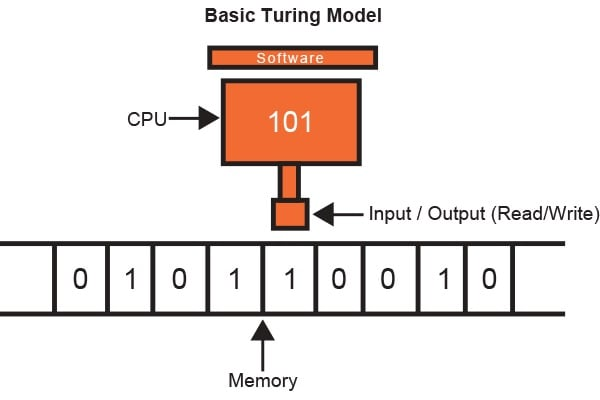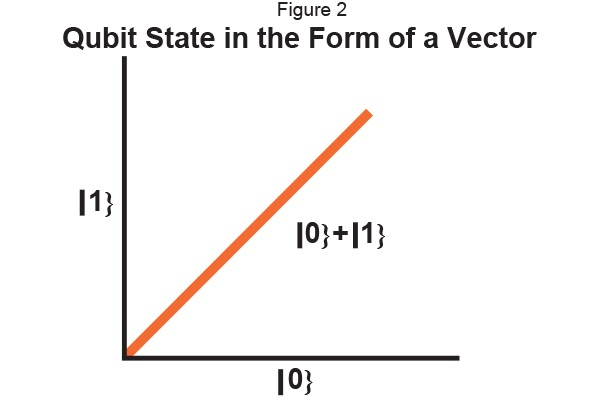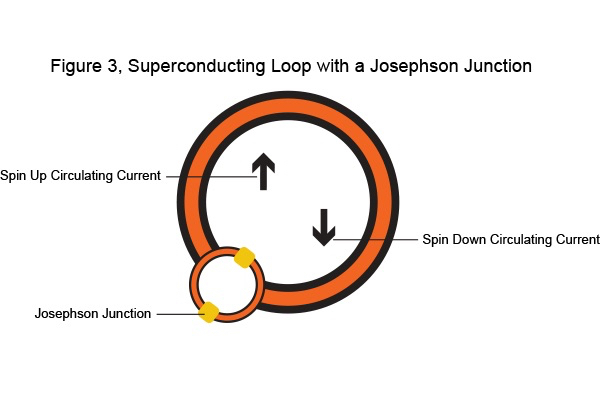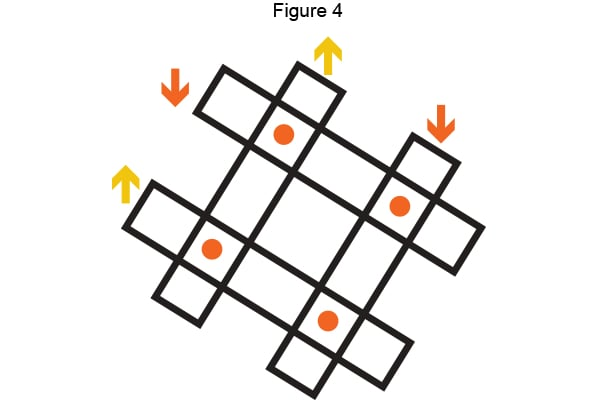Technical Article

# Basic Analysis of a Quantum Processor’s Components

March 17, 2016 by Zabrel Holsman

Learn about the internal components of a Quantum Processor.

### Introduction

First, let’s start by analyzing the concept and components of classical computing. Classic computers will obey the principles of classical physics. A classical computer will perform operations using information stored in the form of bits; whose value is either zero (0) or one (1). Now, when we program a classical computer; we will have a CPU which has an input, an output, and software which regulates the CPU. This is called a Turing Machine, which also happens to be the substructure of your cell phones and laptops computing power. In spite of the relative simplicity, a Turing machine may be constituted to simulate any given computer algorithm’s logic. Unfortunately, even as classical computers have become faster and more concise; they are unable to solve arithmetic like factoring massive integers effectively.In quantum computing, instead of having information stored in the form of bits, we have a new unit called a qubit or quantum bit, which carries quantum information. In a classical system a bit can only be in two positions; either up or down (commonly represented as a zero or one). In quantum computing, the qubit can be in any superposition of both at the same time.

Qubits can be in the in  the given states |0} and |1} (note: 0 and 1 are not always the given values for a qubit, various others may be used but with the same result) as well as any addition of the two; which will yield another valid quantum state x|0} + y|1} where the two variables x and y represent complex numbers. Think of the qubit’s state as being a vector, then the superposed state just becomes additive (See figure 2.) Each additional qubit will then yield two times as many values.  If we were to have 3 qubits we would obtain coefficients for all combinations of 1 and 0 in a 3 digit sequence. (|111},|011}, |001} etc.…)### The Elementary Units of Quantum Computing

In the introduction, we covered how we can represent qubits symbolically as a 0 or 1, as well as a superposition
of both of the states. We will now cover how qubits are constructed as well as their appearance.

In conventional computing, we are using the CMOS transistors to encode bits of information. This is done by regulating the voltage to transistors that are fitted with a bus to determine whether the state is a 0 or 1.

Quantum transistors are somewhat similar, yet vastly different than our current CMOS transistors. Figure 3 shows a quantum transistor known as a Superconducting Quantum Interference Device or a SQUID. Interference refers to the actual electrons, and how they act as waves that create interference patterns to cause quantum effects to occur. This is the basis of quantum computing (basically a quantum transistor.) The electron behaves as a qubit due to the nature of the material called niobium; which is what the gold loop in figure 3 is made of. When the niobium is cooled to reach its critical temperature; it will manifest the qualities of quantum mechanics.

Our classic transistors will encode in two states by regulating voltages. The SQUID will encode the two states into magnetic fields which are designated down or up. The two states are given as -1, +1 in which the qubit can be in superposition of both. This is done by combining the Josephson Effect (or the phenomenon of supercurrent) and the quantization of flux. BCS pairs are tunneled through a weak link (which in this case would be a weak insulating barrier) between the niobium. For each current below a given critical value, a supercurrent will be established between the two superconductors and will yield no voltage across the Josephson junction. Any time a current is larger than the critical value, a voltage will be read across the junction.### The  Programming

The qubits need to be linked together in a fashion that is capable of relaying information. The qubits are attached together by couplers which are also made from superconducting material. When we combine the qubits and couplers together we are capable of creating a programmable structure of quantum mechanics. (See Figure 4.)### Processor Operation

Unfortunately, there are  more components needed to create a functional quantum processor. Much of the structure and circuitry that outlines the qubits are composed of switches that function by the Josephson Effect. This circuitry directs the information from the qubits into various memory components which store the data into a magnetized medium. Each of the qubits is equipped with read-out apparatuses. The read-out will take the vector from the coherent superposition state and project it into a pure zero or one state while losing the phase information. The probability of projection into zero or one state is taken by repeating the procedure many times and averaging the result. These apparatuses will be inoperative while calculations are being made for the qubits to prevent the quantum behavior from being changed. Once calculations have been completed, and after each qubit has established itself into a classical state, the recorded data is converted into a chain of classical bits which can then be read by the user.

##### The structure of the processor is different from the typical silicon processor in that each qubit has individual memory devices instead of large cache areas.

Quantum processing has been speculated to be able to utilize computing power massive orders of magnitude more than our conventional computers. If we take a coherent state qubit system with X qubits then we can superpose 2X different sequences of bits (remember that each additional qubit will yield twice as many values, which is where the 2X comes from.) Now to equate that to conventional computers we take the difference in energy levels of the qubit, in this case, it happens to be in the gigahertz region; which gives us 2X  gigahertz. This means with 20 qubits a quantum processor could process approximately 2^20 operations per second. Based on these figures we can conclude that quantum processors have a substantially greater potential than that of conventional computers.

Recently the Dwave 2X system was manufactured and is considered to be the most powerful quantum computer to date. It happens to operate at 0.015° above absolute zero, and its processor generates no heat. The system is comprised of over 1000 qubits that operate near absolute zero to generate a massive amount of quantum effects. To put this into perspective, the system can search through 2^1000 solutions at once; which is more than every particle in the universe. The Dwave 2X has a rumored list price north of \$15,000,000, and has been released for general availability.

Toward a Superconducting Quantum Computer

Fundamentals of Quantum Computing

Benchmarking a Quantum Annealing Processor with the Time-to-Target Metric (PDF)

• Share• P
PhilTilson March 25, 2016

“...with 20 qubits a quantum processor could process approximately 220 operations per second ... a substantially greater potential than that of conventional computers.”  Huh?

“...the system can search through 21,000 solutions at once; which is more than every particle in the universe.”  What?

Sorry but, like I said, either I am very dim or this article was written by an idiot.

Like.
• Z
Zabrel Holsman March 25, 2016
I appreciate you finding those corrections. Formatting issue caused the superscript to drop. 220 should have been 2^20 and 21000 should have been 2^1000. Thanks, if you find any other numerical or technical corrections feel free to let me know. (No you are not dim, first article so I appreciate the feedback.)
Like.
•NorthGuy March 25, 2016

Either the author doesn’t understand anything about Qantum computers, or he has absolutely no ability to express his thoughts, most likely both.

Like.
• D
dherreiros March 28, 2016

Is not nice people who make rude, morbid or hateful comments, just because they know a little, it costs nothing to be kind…

Like.
•Jblack April 22, 2016

Fantastic article, one error I think is in the last paragraph,  it says “the system can search through 21^000 solutions at once;”  I think that should be 2^1000.

Like.
•tim yb April 22, 2016
You're right, thanks for pointing that out!
Like.